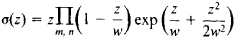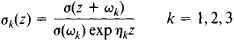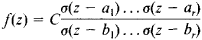# Sigma Functions

(redirected from Divisor function)
Also found in: Wikipedia.
The following article is from The Great Soviet Encyclopedia (1979). It might be outdated or ideologically biased.

## Sigma Functions

entire transcendental functions introduced by K. Weierstrass in his theory of elliptic functions. The principal sigma function (there are four in all) iswhere w = 2mω1+ 2nω21 and ω2 are two numbers such that ω12 is not real) and m and n independently run through all positive and negative integers, other than m = n = 0. The function σ(z) has simple zeros when z = w— that is, at the vertices of the parallelograms forming a regular lattice in the z-plane. These parallelograms are obtained from the parallelogram with vertices at the points 0, 2ω1, 2ω2, and 2(ω1 + ω2) by means of translations along its sides.

The functionσ(z) can be used to determine the Weierstrass zeta function ζ(z) and the Weierstrass elliptic function ℘(z):Let ω3 = – ω1 – ω2 and ζ(ωk) = ηK, where k = 1, 2, 3. The formulas

σ(z + 2wk) = – σ(z) exp [2ηk(z + wk)] k = 1, 2, 3

express the property of quasi periodicity of the function σ(z). The equationsdefine the three remaining sigma functions. We have σ(0) = 0 and σk(0) = 1, k = 1, 2, 3. The function σ(z) is odd, and the other sigma functions are even.

Any elliptic function f(z) with periods 2ω1, and 2ω2 can be rationally expressed in terms of sigma functions by means of the formulawhere C is a constant and a1 …, a, and b1, …, br are, respectively, complete systems of zeros and poles of f(z) satisfying the condition a1 + … + ar = b1 + … + br. Sigma functions are closely related to theta functions.

### REFERENCES

Smirnov, V. I. Kurs vysshei matematiki, 8th ed., vol. 3, part 2. Moscow, 1969.
Hurwitz, A., and R. Courant. Teoriia funktsii. Moscow, 1968. (Translated from German.)
Whittaker, E. T., and G. N. Watson. Kurs sovremennogo analiza, 2nd ed., part 2. Moscow, 1963. (Translated from English.)
The Great Soviet Encyclopedia, 3rd Edition (1970-1979). © 2010 The Gale Group, Inc. All rights reserved.
Mentioned in ?
References in periodicals archive ?
This study applies a growth pattern based on a mathematical model using the convolution sum of the divisor function, as proposed by Kim et al .
For the summatory function (1.6) of the {-dimensional shifted divisor function we have the following theorem.
Let d(m) = [summation over (k|m)] 1 be the divisor function. Using the well--known bounds
References  Laszlo Toth, An order result for the exponential divisor function, Publ.
where d(q) is the divisor function, and (r, s, q) denotes the greatest common divi- sor of r, s and q.
Zhai, On the Smarandache ceil function and the Dirichlet divisor function, Scientia Magna, 4(2008), No.
be the generalized divisor function. Let l [greater than or equal to] 1 and (k, 1) = 1.
Let d(n) denote the Dirichlet divisor function, [S.sub.k] (n) denote the Smarandache cell function, then for 1/4 < [theta] < 1/3, [x.sup.[theta]+2[member of] [less than or equal to] y [less than or equal to] x, we have
In , Gou Su studied the hybrid mean value of Smarandache kn sequence and divisor function [sigma](n), and gave the following theorem:
where d(q - u) is the divisor function. Inserting (3) and (4) into (2), we have
Suppose that 1 [less than or equal to] a [less than or equal to] b are fixed integers, the divisor function d(a, b; k) is defined by
For fixed integers 1 [less than or equal to] a [less than or equal to] b, the divisor function d(a, b; n) is defined by

Site: Follow: Share:
Open / Close